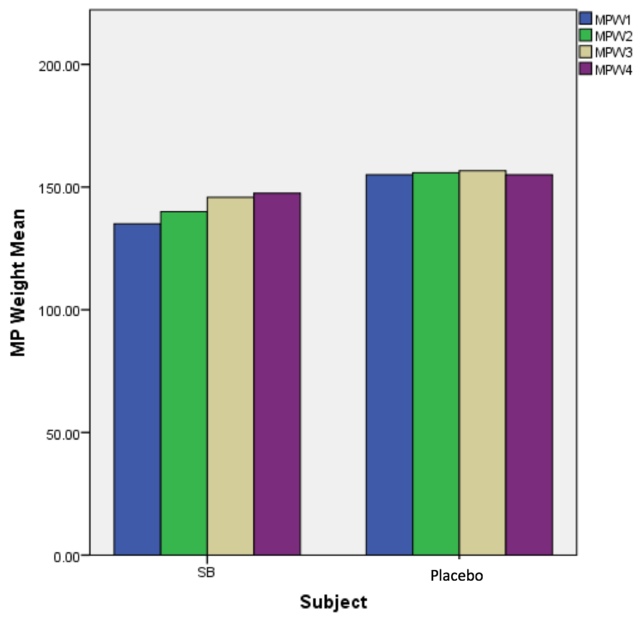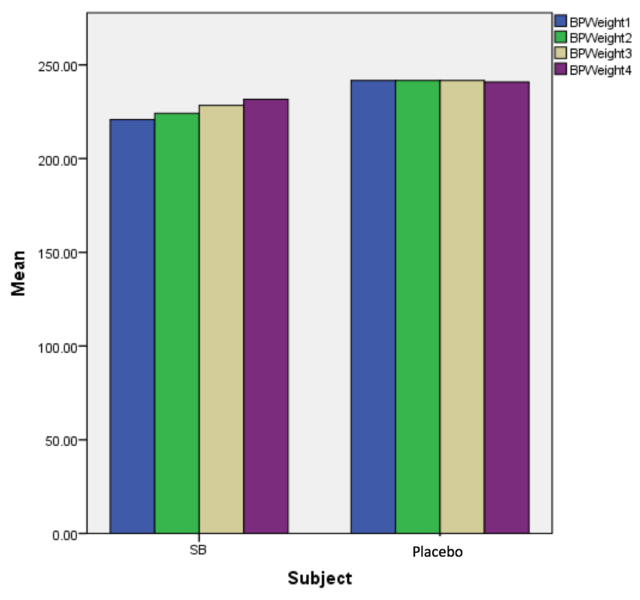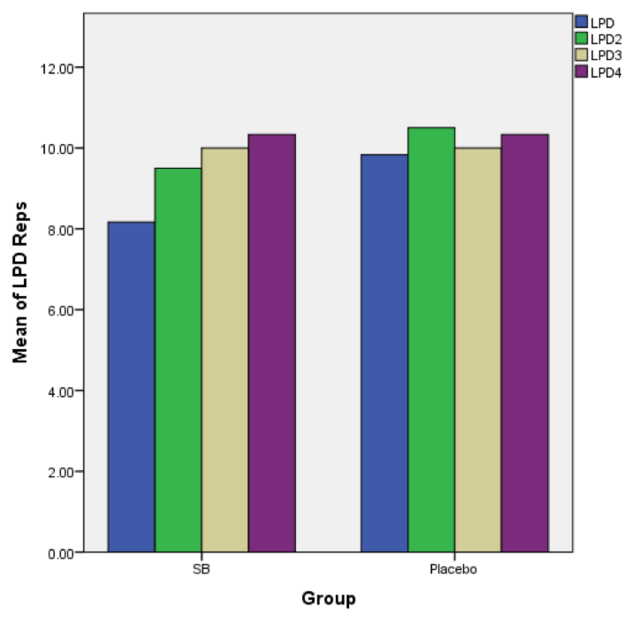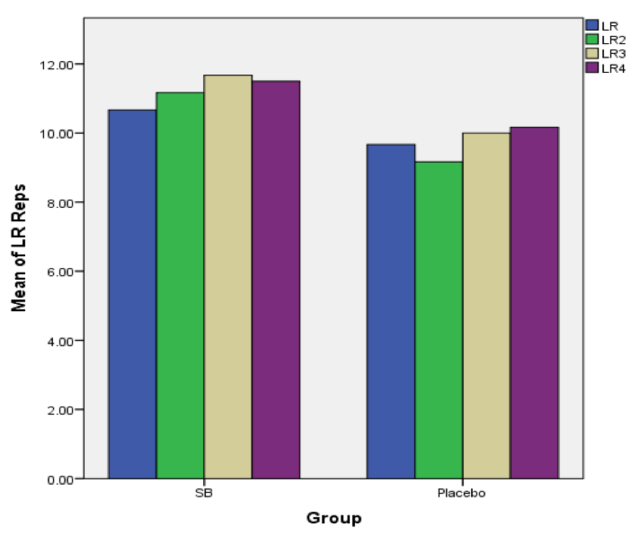# Enhanced Resistance Training Performance via the Neutralization of Lactic Acid with Sodium Bicarbonate

By
2016, Vol. 8 No. 03 | pg. 2/2 |

### Appendix

Figure 1. Group differences as identified by mean weight used in the Back Squat 1RM test in 4 subsequent training sessions. The figure demonstrates that there were increases in weight in the SB group compared to the Control group (P < 0.05).Figure 2. Group differences as identified by mean weight used in the Barbell Row 1RM test in 4 subsequent training sessions. The figure demonstrates that there were increases in weight in the SB group compared to the Control group (P < 0.05).Figure 3. Group differences as identified by mean weight used in the Military Press 1RM test in 4 subsequent training sessions. The figure demonstrates that there were increases in weight in the SB group compared to the Control group (P < 0.05).Figure 4. Group differences as identified by mean weight used in the Bench Press 1RM test in 4 subsequent training sessions. The figure demonstrates that there were increases in weight in the SB group compared to the Control group (P < 0.05).Figure 5. Group differences as identified by mean repetitions performed in the Incline Dumbbell Press endurance test in 4 subsequent training sessions. The figure demonstrates that there were significant increases in repetitions performed in the SB group compared to the Control group (P < 0.05).Figure 6. Group differences as identified by mean repetitions performed in the Lat Pull-down endurance test in 4 subsequent training sessions. The figure demonstrates that there were reasonable increases in repetitions performed in the SB group compared to the Control group (P < 0.05).Figure 7. Group differences as identified by mean repetitions performed in the Leg Press endurance test in 4 subsequent training sessions. The figure demonstrates that there were significant increases in repetitions performed in the SB group compared to the Control group (P < 0.05).Figure 8. Group differences as identified by mean repetitions performed in the Lateral Raise endurance test in 4 subsequent training sessions. The figure did not indicate significant increases in repetitions performed in the SB group compared to the Control group (P > 0.05)Table 1. age (yrs), height (m), weight (lbs), body mass index (BMI) calculations for all 12 subjects

 Descriptive Statistics N Mean Std. Deviation Age 12 20.0000 .95346 Height 12 1.7833 .06746 Weight 12 173.0717 9.90828 BMI 12 25.0833 1.08362 Valid N (listwise) 12

Table 2. Soreness scale values (mean ± SD) for both groups

 Group Statistics Subject N Mean Std. Deviation Std. Error Mean Soreness SB 6 79.8333 6.40052 2.61300 Placebo 6 52.0000 7.37564 3.01109

Table 3. Independent sample t-test for soreness scale

 Independent Samples Test Levene's Test for Equality of Variances t-test for Equality of Means F Sig. t df Sig. (2-tailed) Mean Difference Std. Error Difference 95% Confidence Interval of the Difference Lower Upper Soreness Equal variances assumed .703 .421 6.981 10 .000 27.83333 3.98678 18.95023 36.71644 Equal variances not assumed 6.981 9.805 .000 27.83333 3.98678 18.92628 36.74039

Table 4. Intensity scale values (mean ± SD) for both groups

 Group Statistics Subject N Mean Std. Deviation Std. Error Mean Intensity SB 6 86.6667 2.94392 1.20185 Placebo 6 59.0000 5.83095 2.38048

Table 5. Independent sample t-test for intensity scale

 Independent Samples Test Levene's Test for Equality of Variances t-test for Equality of Means F Sig. t df Sig. (2-tailed) Mean Difference Std. Error Difference 95% Confidence Interval of the Difference Lower Upper Intensity Equal variances assumed .984 .345 10.375 10 .000 27.66667 2.66667 21.72496 33.60837 Equal variances not assumed 10.375 7.394 .000 27.66667 2.66667 21.42840 33.90493

Table 6. Improvement scale values (mean ± SD) for both groups

 Group Statistics Subject N Mean Std. Deviation Std. Error Mean Improvement SB 6 85.0000 4.19524 1.71270 Placebo 6 47.6667 4.92612 2.01108

Table 7. Independent sample t-test for improvement scale

 Independent Samples Test Levene's Test for Equality of Variances t-test for Equality of Means F Sig. t df Sig. (2-tailed) Mean Difference Std. Error Difference 95% Confidence Interval of the Difference Lower Upper Improvement Equal variances assumed .018 .897 14.133 10 .000 37.33333 2.64155 31.44760 43.21907 Equal variances not assumed 14.133 9.753 .000 37.33333 2.64155 31.42730 43.23936